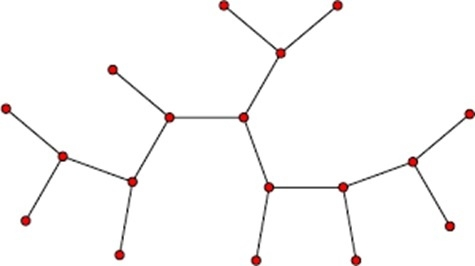# Check if a given graph is tree or not

In this problem, one undirected graph is given, we have to check the graph is tree or not. We can simply find it by checking the criteria of a tree. A tree will not contain a cycle, so if there is any cycle in the graph, it is not a tree.We can check it using another approach, if the graph is connected and it has V-1 edges, it could be a tree. Here V is the number of vertices in the graph.

## Input and Output

Input:
0 0 0 0 1
0 0 0 0 1
0 0 0 0 1
0 0 0 0 1
1 1 1 1 0

Output:
The Graph is a tree

## Algorithm

isCycle(u, visited, parent)

Input: The start vertex u, the visited list to mark visited or not, the parent vertex.

Output: True if there is a cycle in the graph.

Begin
mark u as visited
for all vertex v which are adjacent with u, do
if v is visited, then
if isCycle(v, visited, u) = true, then
return true
else if v ≠ parent, then
return true
done
return false
End

isTree(graph)

Input: The undirected graph.

Output: True when the graph is a tree.

Begin
define a visited array to mark which node is visited or not
initially mark all node as unvisited
if isCycle(0, visited, φ) is true, then //the parent of starting vertex is null
return false
if the graph is not connected, then
return false
return true otherwise
End

## Example

#include<iostream>
#define NODE 5
using namespace std;

int graph[NODE][NODE] = {
{0, 1, 1, 1, 0},
{1, 0, 1, 0, 0},
{1, 1, 0, 0, 0},
{1, 0, 0, 0, 1},
{0, 0, 0, 1, 0}
};

bool isCycle(int u, bool visited[], int parent) {
visited[u] = true;    //mark v as visited
for(int v = 0; v<NODE; v++) {
if(graph[u][v]) {
if(!visited[v]) {     //when the adjacent node v is not visited
if(isCycle(v, visited, u)) {
return true;
}
} else if(v != parent) {    //when adjacent vertex is visited but not parent
return true;    //there is a cycle
}
}
}
return false;
}

bool isTree() {
bool *vis = new bool[NODE];

for(int i = 0; i<NODE; i++)
vis[i] = false;    //initialize as no node is visited

if(isCycle(0, vis, -1))    //check if there is a cycle or not
return false;

for(int i = 0; i<NODE; i++) {
if(!vis[i])    //if there is a node, not visited by traversal, graph is not connected
return false;
}
return true;
}

int main() {
if(isTree())
cout << "The Graph is a Tree.";
else
cout << "The Graph is not a Tree.";
}

## Output

The Graph is a Tree.